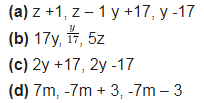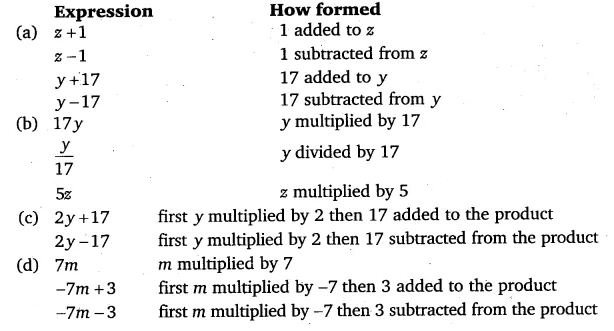# Identify the operations (addition, subtraction, division, multiplication)

Identify the operations (addition, subtraction, division, multiplication) in forming the following expressions and tell how the expressions have been formed: